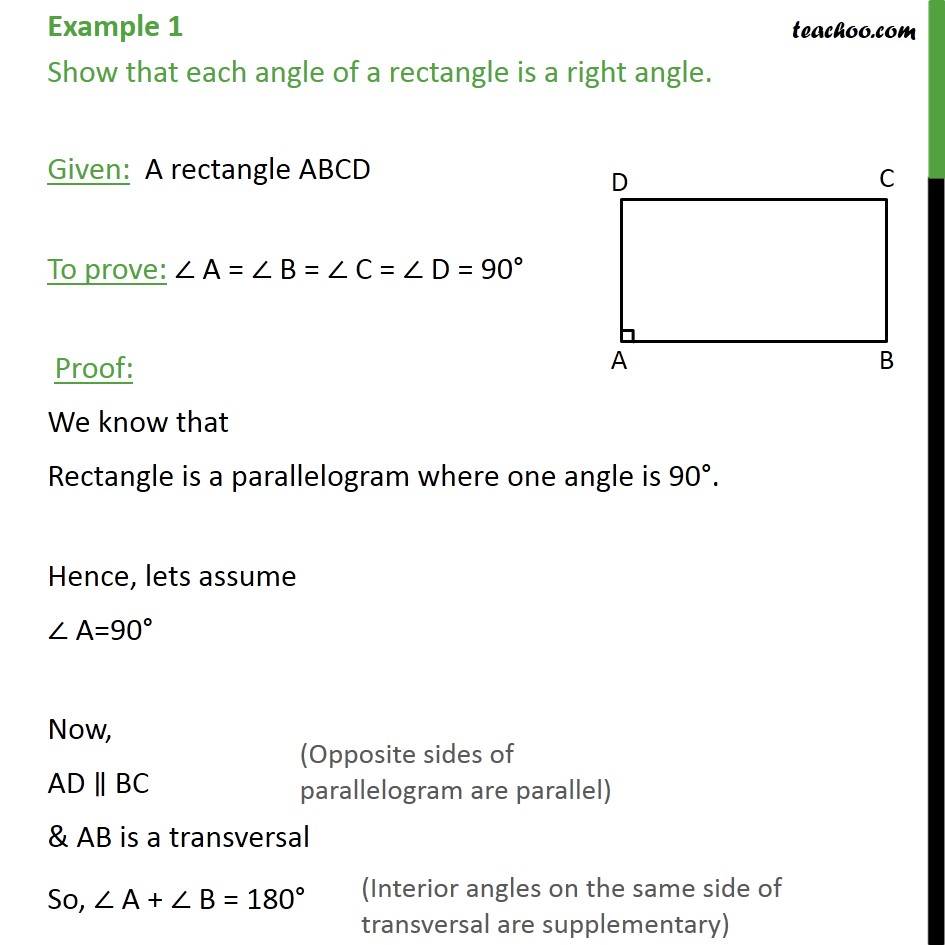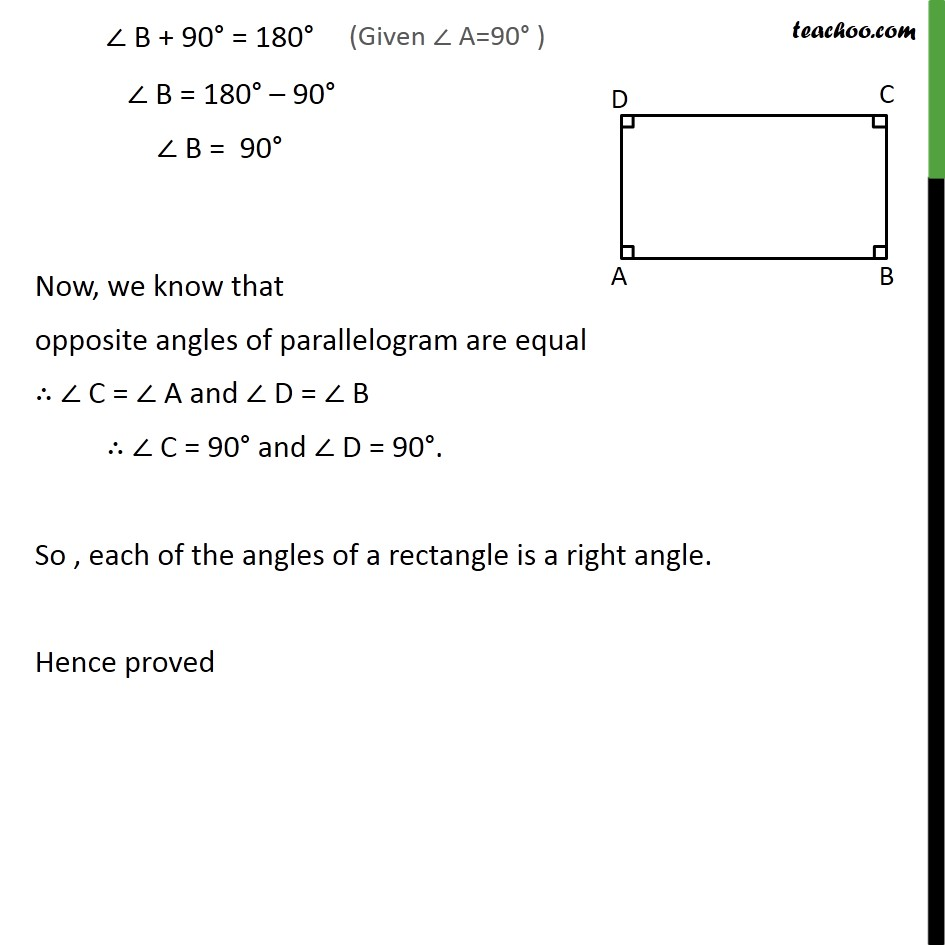Opposite angles of parallelogram

Concept wiseLearn in your speed, with individual attention - Teachoo Maths 1-on-1 Class

### Transcript

Example 1 Show that each angle of a rectangle is a right angle. Given: A rectangle ABCD To prove: ∠ A = ∠ B = ∠ C = ∠ D = 90° Proof: We know that Rectangle is a parallelogram where one angle is 90°. Hence, lets assume ∠ A=90° Now, AD ∥ BC & AB is a transversal So, ∠ A + ∠ B = 180° ∠ B + 90° = 180° ∠ B = 180° – 90° ∠ B = 90° Now, we know that opposite angles of parallelogram are equal ∴ ∠ C = ∠ A and ∠ D = ∠ B ∴ ∠ C = 90° and ∠ D = 90°. So , each of the angles of a rectangle is a right angle. Hence proved